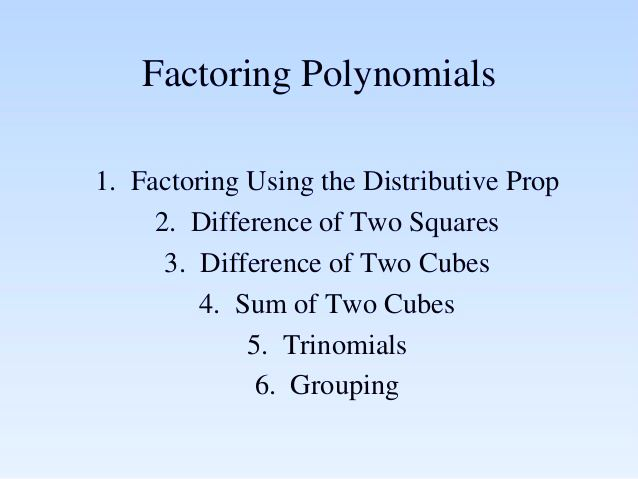# Factoring Polynomials: How To Factorise

Factoring polynomials is the reverse procedure of multiplication of factors of polynomials. An expression of the form axn + bxn-1 + cxn-2 + ….+kx+ l, where each variable has a constant accompanying it as its coefficient is called a polynomial of degree ‘n’ in variable x. Thus, a polynomial is an expression in which a combination of a constant and a variable is separated by an addition or a subtraction sign. Till now we have learnt about calculating zeroes of polynomials. Zeros of polynomials, when represented in the form of another linear polynomial are known as factors of polynomials. After factorisation of a given polynomial, if we divide the polynomial with any of its factors, the remainder will be zero. Also, in this process, we factor the polynomial by finding its greatest common factor. Now let us learn how to factorise polynomials here with examples.## Factorisation of Polynomial

The process of finding factors of a given value is called factorisation. Factors are the integers which are multiplied to produce an original number. For example, the factors of 18 are 2, 3, 6, 9 and 18, such as;

18 = 2 x 9

18 = 2 x 3 x 3

18 = 3 x 6

Similarly, in the case of polynomials, the factors are the polynomials which are multiplied to produce the original polynomial. For example, the factors of x2 + 5x + 6 is (x + 2) (x + 3). When we multiplied both x +2 and x+3, then the original polynomial is generated. After factorisation, we can also find the zeros of the polynomials. In this case, x = -2 and x = -3.

### Greatest Common Factor

We have to find out the greatest common factor, of the given polynomial to factorise it. This process is nothing but a type of reverse procedure of distributive law, such as;

p( q + r) = pq + pr

But in the case of factorisation, it is just an inverse process;

pq + pr = p(q + r)

where p is the greatest common factor.

### Factor theorem

For a polynomial p(x) of degree greater than or equal to one,

1. x-a is a factor of p(x), if p(a) = 0
2. if p(a) = 0, x-a is a factor of p(x)

Where ‘a’ is a real number

## Factoring Polynomials Examples

Question 1: Check whether x+3 is a factor of x3 + 3x2 + 5x +15?

Solution: Let, q(x) = x + 3, for calculating zero of this polynomial

x + 3 = 0

=> x = -3

Now, p(x) = x3 + 3x2 + 5x +15, let us check the value of this polynomial for x = -3,

p(-3) = (-3)3 + 3 (-3)2 + 5(-3) + 15 = -27 + 27 – 15 + 15 = 0

As, p(-3) = 0,  x+3 is a factor of x3 + 3x2 + 5x +15.

Question 2: Factorize x2 + 5x + 6.

Solution: Let us try factorizing this polynomial using splitting the middle term method

Factoring polynomials by splitting the middle term:

In this technique we need to find two terms ‘a’ and ‘b’ such that a + b =5 and ab = 6. On solving this we obtain, a = 3 and b = 2

Thus the above expression can be written as,

x2 + 3x + 2x + 6 = x(x + 3) + 2(x + 3) = (x + 3)(x + 2)

Thus, x+3 and x+2 are the factors of the polynomial x2 + 5x + 6.